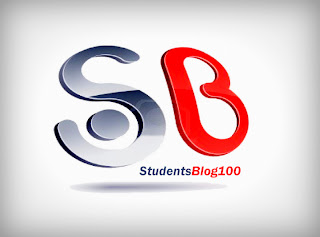CS6012 SOFT COMPUTING SYLLABUS FOR 8TH SEM CSE REGULATION 2013 - Anna University Internal marks 2018
ANNA UNIVERSITY CSE SYLLABUS
CS6012 SOFT COMPUTING SYLLABUS
8TH SEMESTER CSE
REGULATION 2013CS6012 SOFT COMPUTING SYLLABUS
OBJECTIVES:
The student should be made to:

-> Learn the various soft computing frame works.
-> Be familiar with design of various neural networks.
-> Be exposed to fuzzy logic.
-> Learn genetic programming.
-> Be exposed to hybrid systems.

UNIT I INTRODUCTION
Artificial neural network: Introduction, characteristics- learning methods – taxonomy – Evolution of neural networks- basic models - important technologies - applications. Fuzzy logic: Introduction - crisp sets- fuzzy sets - crisp relations and fuzzy relations: cartesian product of relation - classical relation, fuzzy relations, tolerance and equivalence relations, non-iterative fuzzy sets. Genetic algorithm- Introduction - biological background - traditional optimization and search techniques - Genetic basic concepts.

UNIT II NEURAL NETWORKS
McCulloch-Pitts neuron - linear separability - hebb network - supervised learning network: perceptron networks - adaptive linear neuron, multiple adaptive linear neuron, BPN, RBF, TDNN- associative memory network: auto-associative memory network, hetero-associative memory network, BAM, hopfield networks, iterative autoassociative memory network & iterative associative memory network – unsupervised learning networks: Kohonen self organizing feature maps, LVQ – CP networks, ART network.

UNIT III FUZZY LOGIC
Membership functions: features, fuzzification, methods of membership value assignments- Defuzzification: lambda cuts - methods - fuzzy arithmetic and fuzzy measures: fuzzy arithmetic -extension principle - fuzzy measures - measures of fuzziness -fuzzy integrals - fuzzy rule base and approximate reasoning : truth values and tables, fuzzy propositions, formation of rules-decomposition of rules, aggregation of fuzzy rules, fuzzy reasoning-fuzzy inference systems-overview of fuzzy expert system-fuzzy decision making.

UNIT IV GENETIC ALGORITHM
Genetic algorithm and search space - general genetic algorithm – operators - Generational cycle - stopping condition – constraints - classification - genetic programming – multilevel optimization – real life problem- advances in GA.

UNIT V HYBRID SOFT COMPUTING TECHNIQUES & APPLICATIONS
Neuro-fuzzy hybrid systems - genetic neuro hybrid systems - genetic fuzzy hybrid and fuzzy genetic hybrid systems - simplified fuzzy ARTMAP - Applications: A fusion approach of multispectral images with SAR, optimization of traveling salesman problem using genetic algorithm approach, soft computing based hybrid fuzzy controllers.

TOTAL: 45 PERIODS

OUTCOMES:
Upon completion of the course, the student should be able to:

-> Apply various soft computing frame works.
-> Design of various neural networks.
-> Use fuzzy logic.
-> Apply genetic programming.
-> Discuss hybrid soft computing.

TEXT BOOKS:
1. J.S.R.Jang, C.T. Sun and E.Mizutani, “Neuro-Fuzzy and Soft Computing”, PHI / Pearson Education 2004.
2. S.N.Sivanandam and S.N.Deepa, "Principles of Soft Computing", Wiley India Pvt Ltd, 2011.

REFERENCES:
1. S.Rajasekaran and G.A.Vijayalakshmi Pai, "Neural Networks, Fuzzy Logic and Genetic Algorithm: Synthesis & Applications", Prentice-Hall of India Pvt. Ltd., 2006.
2. George J. Klir, Ute St. Clair, Bo Yuan, “Fuzzy Set Theory: Foundations and Applications” Prentice Hall, 1997.
3. David E. Goldberg, “Genetic Algorithm in Search Optimization and Machine Learning” Pearson Education India, 2013.
4. James A. Freeman, David M. Skapura, “Neural Networks Algorithms, Applications, and Programming Techniques, Pearson Education India, 1991.
5. Simon Haykin, “Neural Networks Comprehensive Foundation” Second Edition, Pearson Education, 2005.